Register for Maths, Science, English, Reasoning Olympiad Exams Register here | Book Free Demo for Live Olympiad Classes here | Check Olympiad Exam Dates here | Buy Practice Papers for IMO, IOM, HEO, IOEL etc here | Login here to participate in all India free mock test on every Saturday

# Weight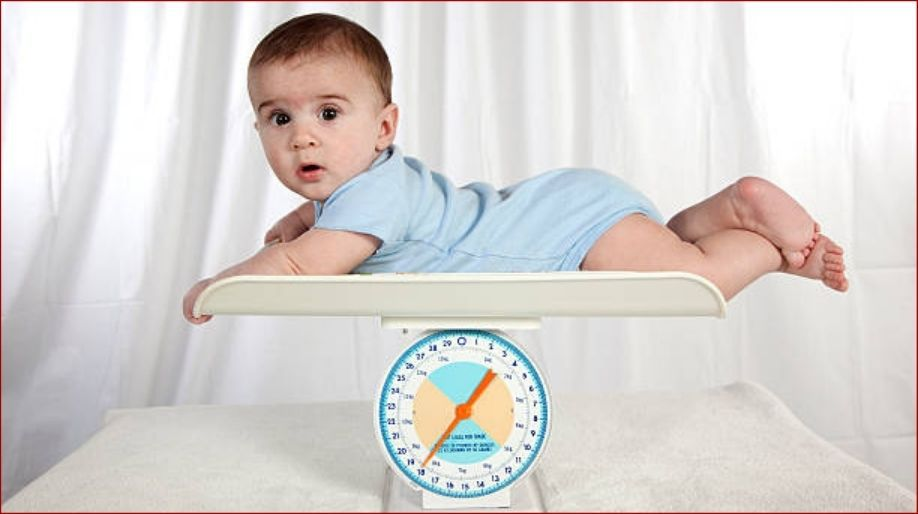What is weight? Weight is the amount of matter present in a body. It is denoted by W. Do you know your weight? Have you asked your father’s weight? Ask him how to measure it.Heavy body

A body which has more weight is called heavy. All things which are large in size and are hard are mostly heavy.

Examples: Elephant, bus, train, ship, scooter, car, etc.

Light body

A body which has less weight is called light. All things which are light in weight and easy to handle and pick our light bodies.

Example- Paper, feather, pencil, leaf, etc.

Let’s understand the concept of a heavy and light body by pictures.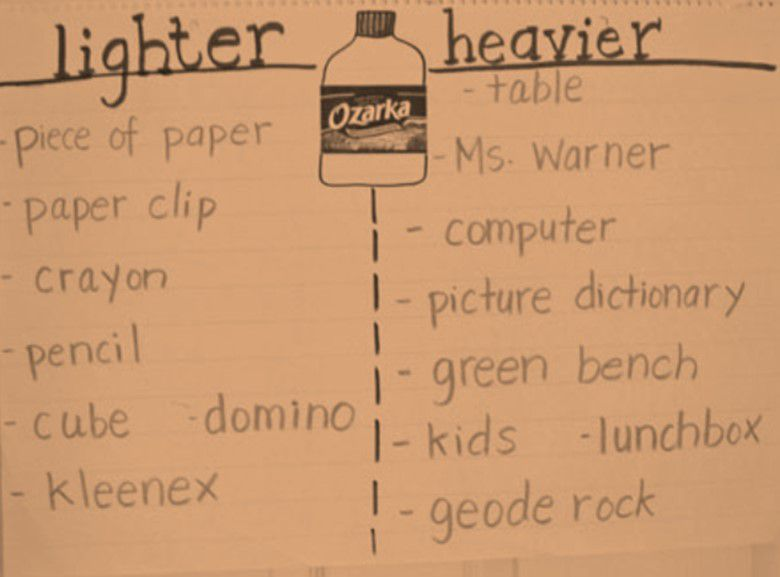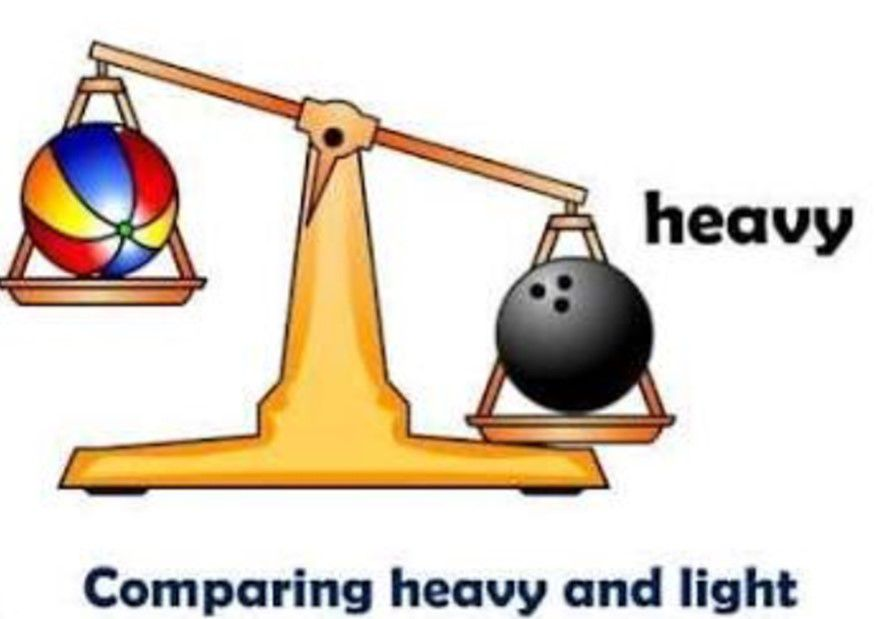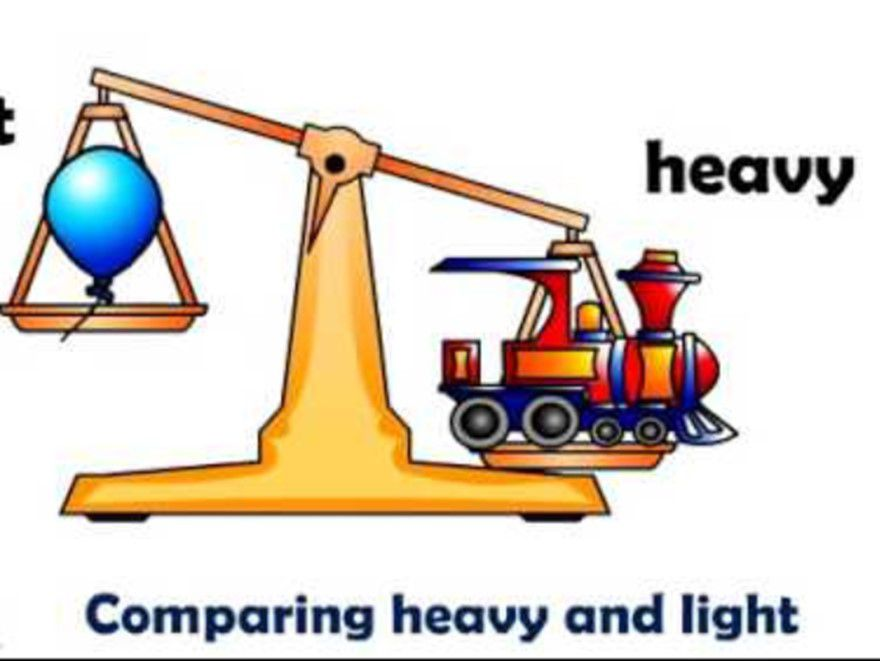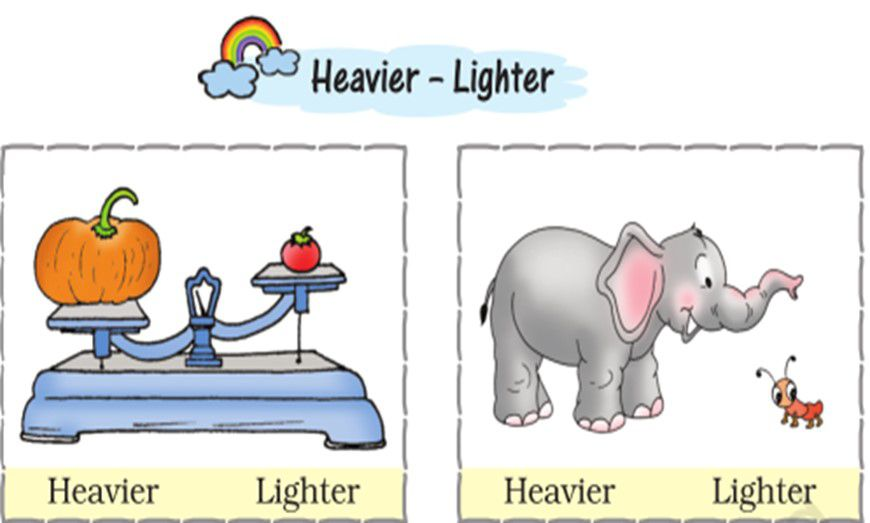Measurement of Weight

Weight is the measurement of the heaviness of the body. Have you measured your weight? Do you want to know how weight is measured? Weight is measured by weighing machine and is represented in gram and kilogram. By measuring weight you can tell which body is heavy and which is light. Let us understand about the measurement of weight with figures in fun.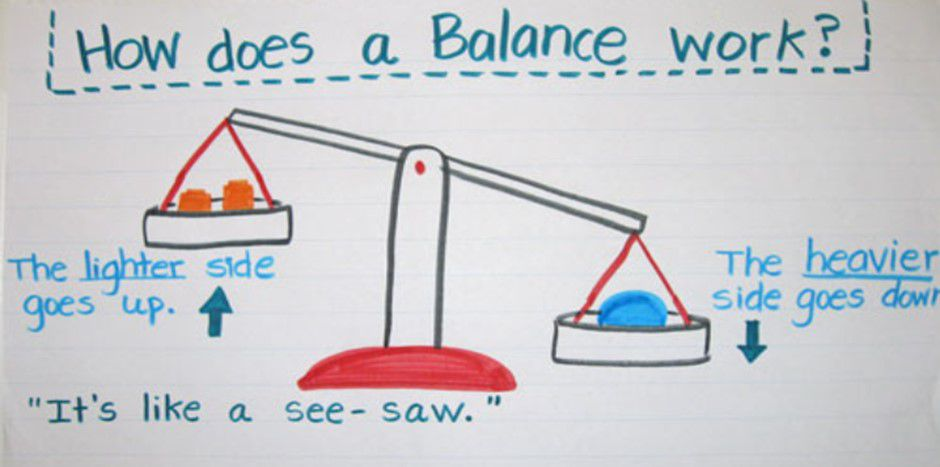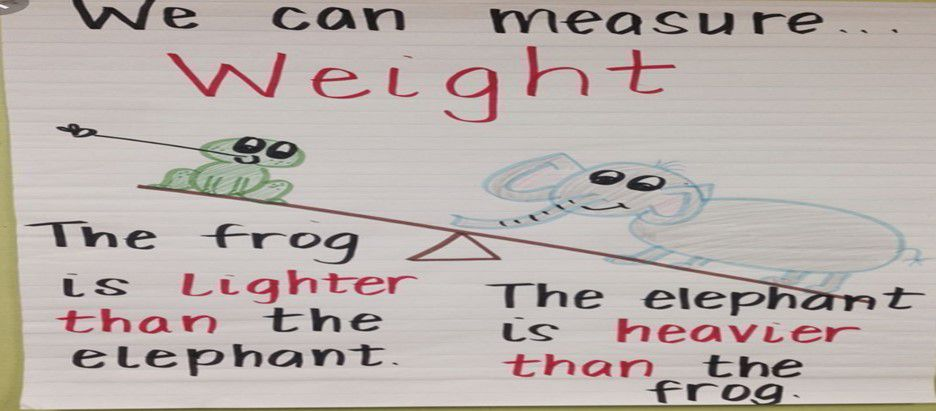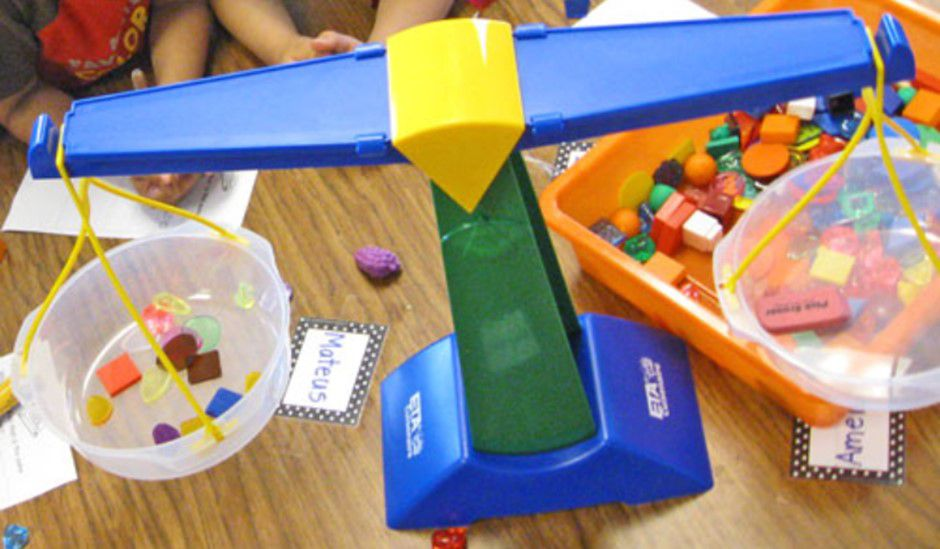Solved examples to identify either body is heavy or light.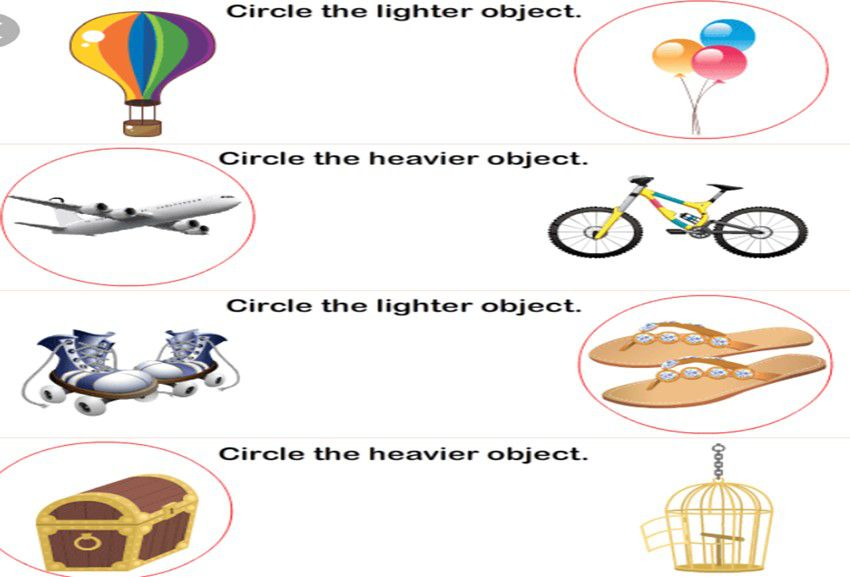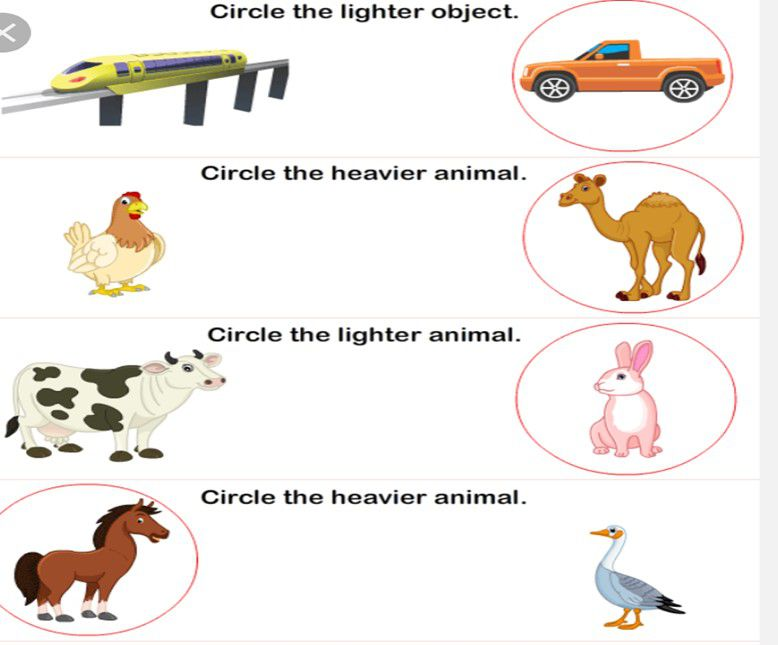Practice Questions on light and heavy body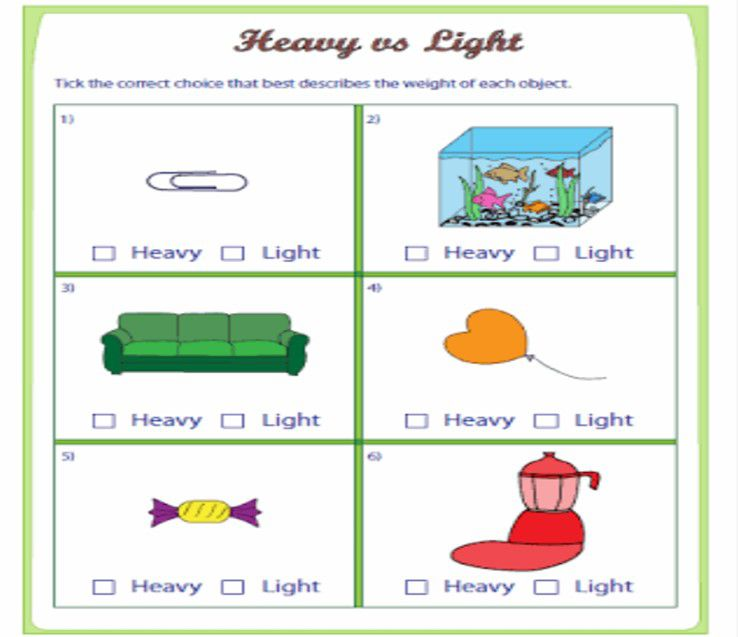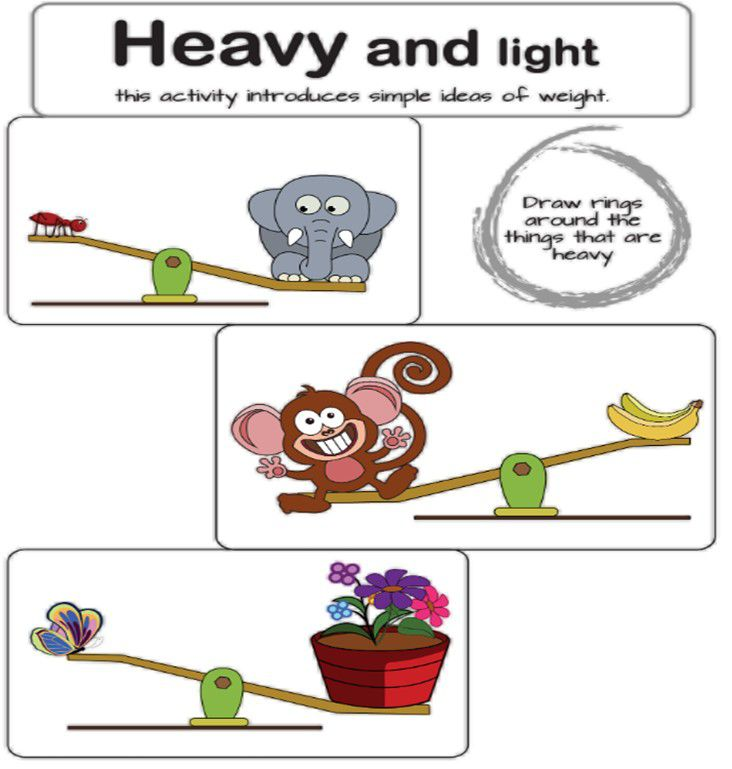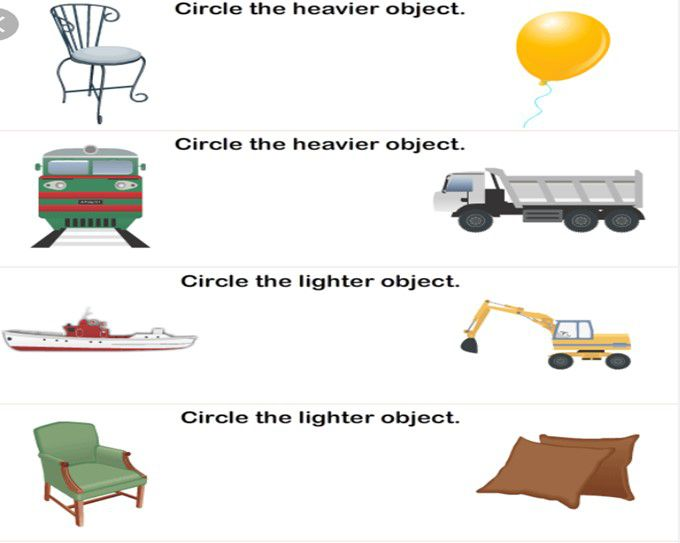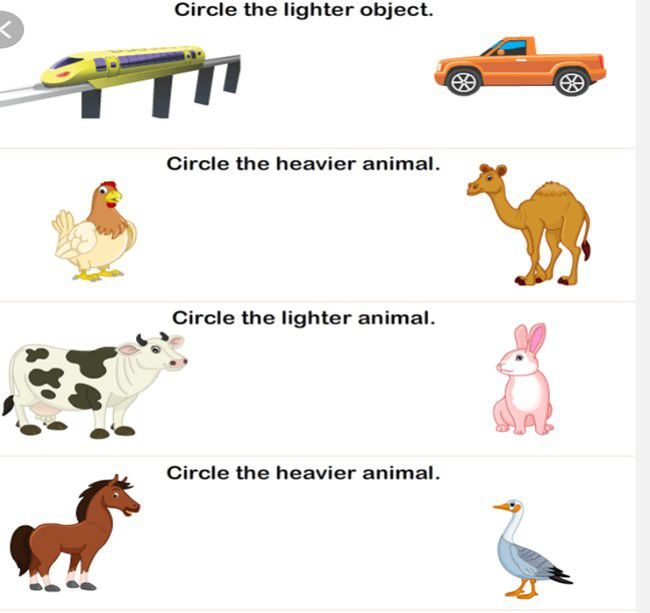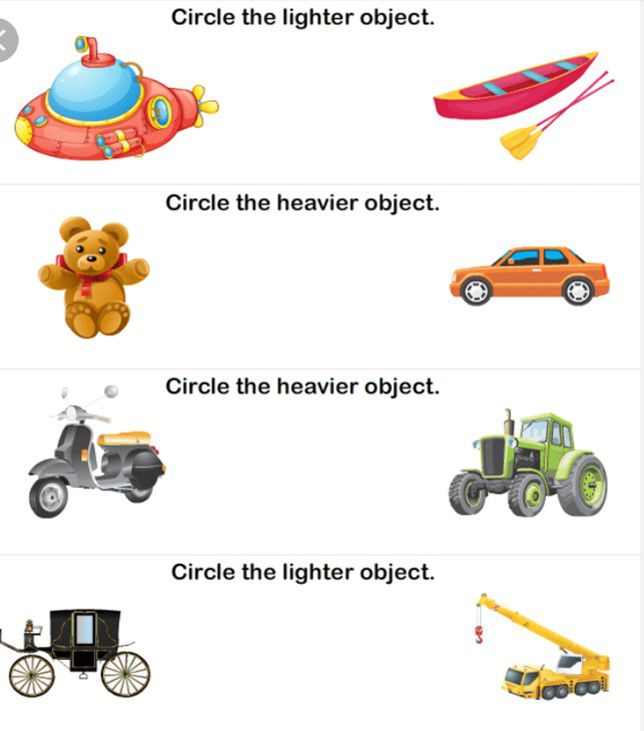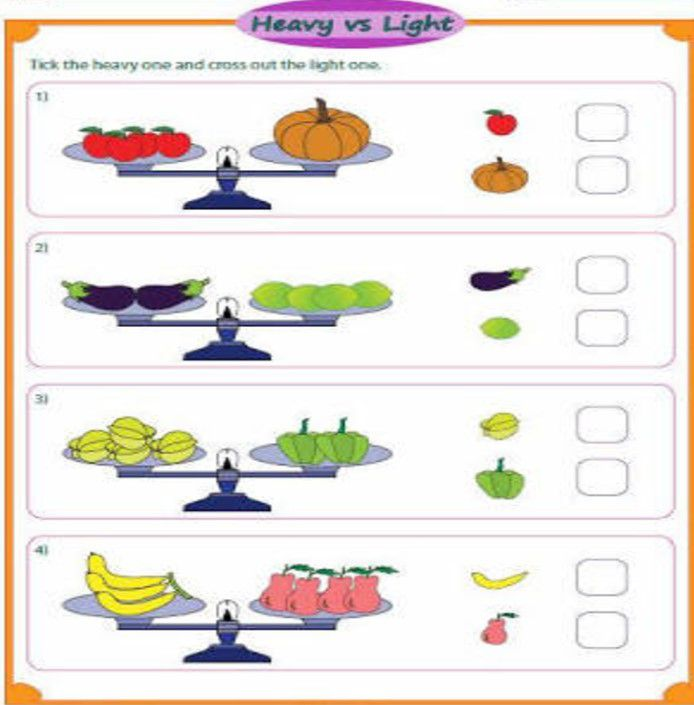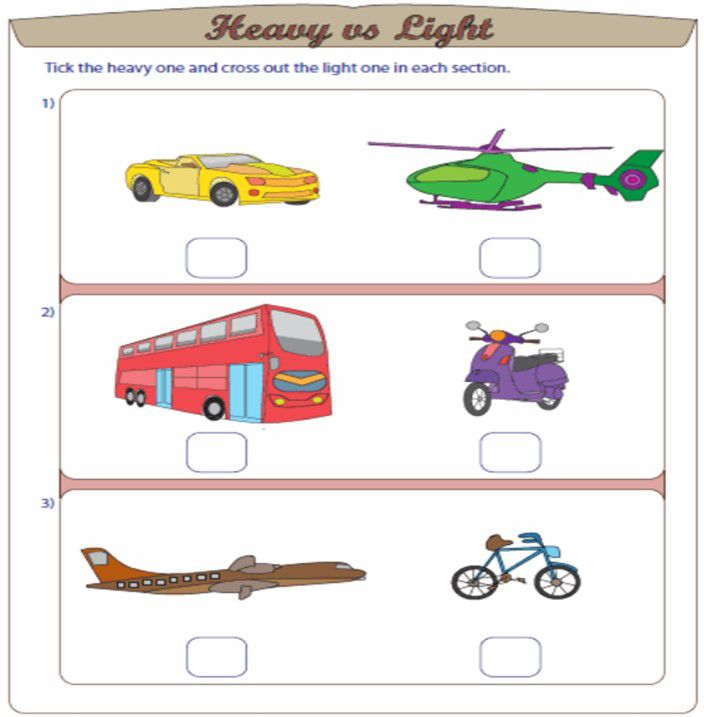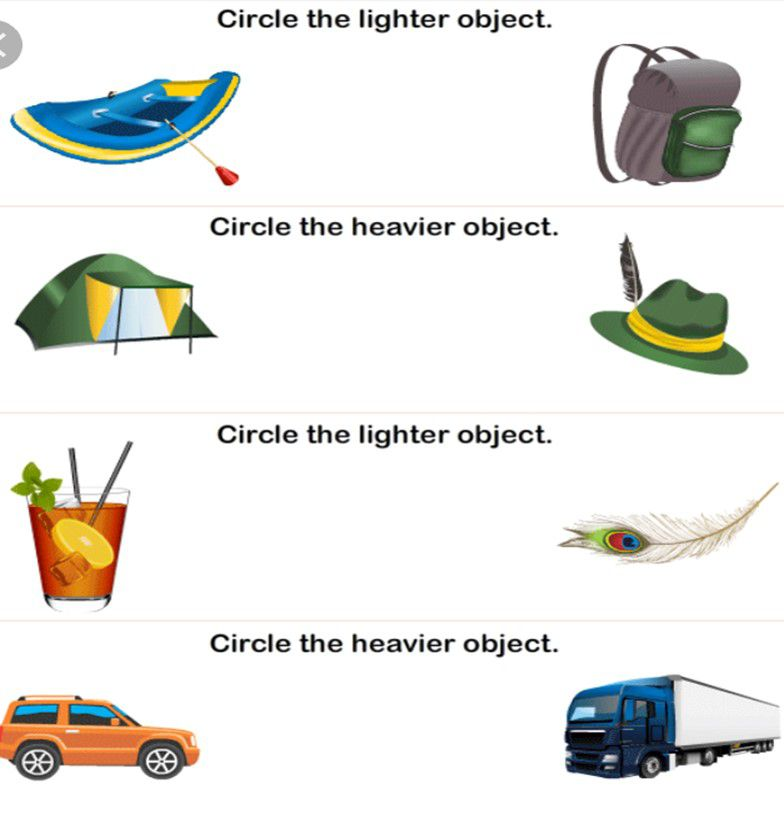Recap

• Weight is the amount of matter present in a body.
• Bodies which have more weight are called heavy bodies.
• Bodies which have less weight are called light bodies.

## Quiz for Weight

 Q.1 Find the odd one out: a) meter b) kilogram c) gram d) centigram
 Q.2 Which among the following is a unit to measure weight? a) second b) meter c) kilogram d) centimeter
 Q.3 The above image represent how many weight?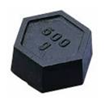a) 500 gm b) 250 gm c) 850 gm d) 1 kg
 Q.4 What do you call to the object shown in the above image?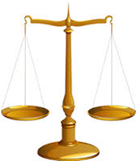a) weighing machine b) stone measure c) weighing balance d) balance body
 Q.5 While weighing an object it read as 5kg, what will be its value in gram? a) 500 gm b) 5 gm c) 5000gm d) 50 gm
 Q.6 While weighing an object it read as 20 gm, so what is its weight in kg? a) .002kg b) 0.00002 kg c) .02kg d) 2 kg
 Q.7 For weighting this object shown in figure, which unit of measurement should be used?( car)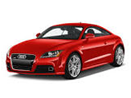a) pound b) gram c) ton d) ounce
 Q.8 1 Ounce is equal to how many milligrams? a) 283495 milligram b) 28394.5 milligram c) 28349.5 milligram d) 2834.95 milligram
 Q.9 What is the weight shown in the image in pound?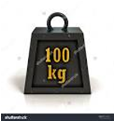a) 220 pounds b) 110 pound c) 240 pound d) 50 pound
 Q.10 Represent the following given weight in Hectogram? 100 g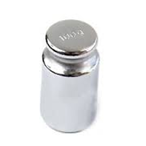a) 10 Hectogram b) 1 hectogram c) 16 Hectogram d) 13 Hectogram